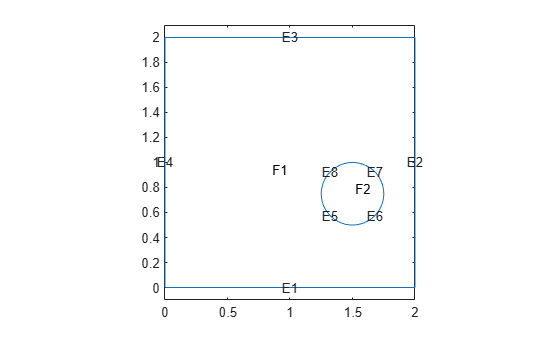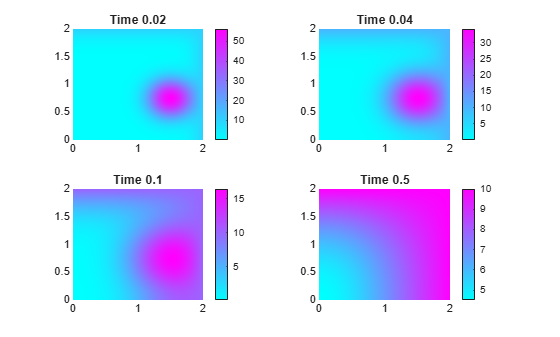# TimeDependentResults

Time-dependent PDE solution and derived quantities

## Description

A `TimeDependentResults` object contains the solution of a PDE and its gradients in a form convenient for plotting and postprocessing.

• A `TimeDependentResults` object contains the solution and its gradient calculated at the nodes of the triangular or tetrahedral mesh, generated by `generateMesh`.

• Solution values at the nodes appear in the `NodalSolution` property.

• The solution times appear in the `SolutionTimes` property.

• The three components of the gradient of the solution values at the nodes appear in the `XGradients`, `YGradients`, and `ZGradients` properties.

• The array dimensions of `NodalSolution`, `XGradients`, `YGradients`, and `ZGradients` enable you to extract solution and gradient values for specified time indices, and for the equation indices in a PDE system.

To interpolate the solution or its gradient to a custom grid (for example, specified by `meshgrid`), use `interpolateSolution` or `evaluateGradient`.

## Creation

There are several ways to create a `TimeDependentResults` object:

• Solve a time-dependent problem using the `solvepde` function. This function returns a PDE solution as a `TimeDependentResults` object. This is the recommended approach.

• Solve a time-dependent problem using the `parabolic` or `hyperbolic` function. Then use the `createPDEResults` function to obtain a `TimeDependentResults` object from a PDE solution returned by `parabolic` or `hyperbolic`. Note that `parabolic` and `hyperbolic` are legacy functions. They are not recommended for solving PDE problems.

## Properties

expand all

Finite element mesh, returned as a FEMesh Properties object.

Solution values at the nodes, returned as a vector or array. For details about the dimensions of `NodalSolution`, see Dimensions of Solutions, Gradients, and Fluxes.

Data Types: `double`
Complex Number Support: Yes

Solution times, returned as a real vector. `SolutionTimes` is the same as the `tlist` input to `solvepde`, or the `tlist` input to the legacy `parabolic` or `hyperbolic` solvers.

Data Types: `double`

x-component of the gradient at the nodes, returned as a vector or array. For details about the dimensions of `XGradients`, see Dimensions of Solutions, Gradients, and Fluxes.

Data Types: `double`
Complex Number Support: Yes

y-component of the gradient at the nodes, returned as a vector or array. For details about the dimensions of `YGradients`, see Dimensions of Solutions, Gradients, and Fluxes.

Data Types: `double`
Complex Number Support: Yes

z-component of the gradient at the nodes, returned as a vector or array. For details about the dimensions of `ZGradients`, see Dimensions of Solutions, Gradients, and Fluxes.

Data Types: `double`

## Object Functions

 `evaluateCGradient` Evaluate flux of PDE solution `evaluateGradient` Evaluate gradients of PDE solutions at arbitrary points `interpolateSolution` Interpolate PDE solution to arbitrary points

## Examples

collapse all

Solve a parabolic problem with 2-D geometry.

Create and view the geometry: a square with a circular subdomain.

```% Square centered at (1,1) rect1 = [3;4;0;2;2;0;0;0;2;2]; % Circle centered at (1.5,0.5) circ1 = [1;1.5;.75;0.25]; % Append extra zeros to the circle circ1 = [circ1;zeros(length(rect1)-length(circ1),1)]; gd = [rect1,circ1]; ns = char('rect1','circ1'); ns = ns'; sf = 'rect1+circ1'; [dl,bt] = decsg(gd,sf,ns); pdegplot(dl,"EdgeLabels","on","FaceLabels","on") axis equal ylim([-0.1,2.1])```Include the geometry in a PDE model.

```model = createpde(); geometryFromEdges(model,dl);```

Set boundary conditions that the upper and left edges are at temperature 10.

`applyBoundaryCondition(model,"dirichlet","Edge",[2,3],"u",10);`

Set initial conditions that the square region is at temperature 0, and the circle is at temperature 100.

```setInitialConditions(model,0); setInitialConditions(model,100,"Face",2);```

Define the model coefficients.

`specifyCoefficients(model,"m",0,"d",1,"c",1,"a",0,"f",0);`

Solve the problem for times 0 through 1/2 in steps of 0.01.

```generateMesh(model,"Hmax",0.05); tlist = 0:0.01:0.5; results = solvepde(model,tlist);```

Plot the solution for times 0.02, 0.04, 0.1, and 0.5.

```sol = results.NodalSolution; subplot(2,2,1) pdeplot(model,"XYData",sol(:,3)) title("Time 0.02") subplot(2,2,2) pdeplot(model,"XYData",sol(:,5)) title("Time 0.04") subplot(2,2,3) pdeplot(model,"XYData",sol(:,11)) title("Time 0.1") subplot(2,2,4) pdeplot(model,"XYData",sol(:,51)) title("Time 0.5")```## Version History

Introduced in R2016a